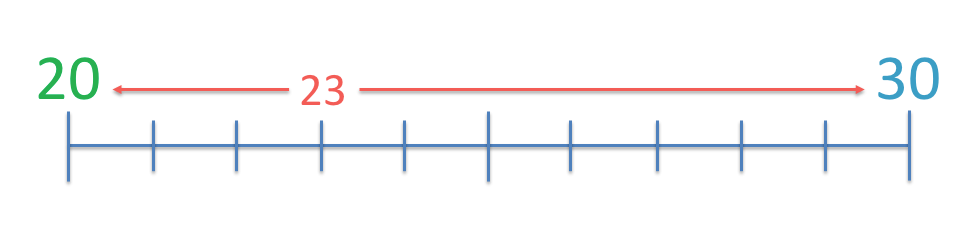# Comparing Number Sentences using <, > and =

Comparing Number Sentences using <, > and =• Here we need to fill in the missing symbol between 30 + 10 and 80.
• We work out the addition sum on one side of the missing symbol.
• 30 + 10 = 40.
• We can see that 40 is to the left of 80 on the number line.
• 40 is less than 80 and so we use the less than sign, ‘<'.
• We say that 30 + 10 < 80.
• The less than sign always points at the smaller number and opens up to the larger number.

Work out the sum on one side of the missing symbol box.

Insert a less-than or greater-than symbol which points at the smaller value side.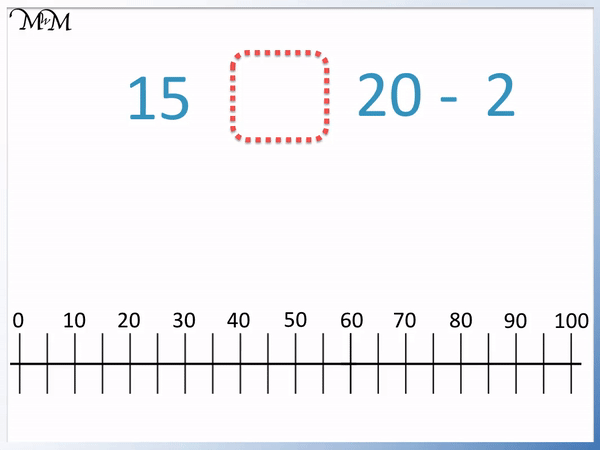• We first work out the side which has the subtraction sum.
• 20 – 2 = 18.
• 18 is further to the right of 15 on the number line.
• 18 is greater than 15 and so 15 is less than 18.
• We use the less-than symbol, ‘<' to say that 15 is less than 18.
• We write 15 < 18 and so 15 < 20 - 2.
• The less-than sign always points to the smaller value and opens to the larger value.# How to Compare Number Sentences using Greater-Than and Less-Than Signs

We use less-than and greater-than signs to compare numbers.

‘<' is the less-than symbol.

‘>’ is the greater-than symbol.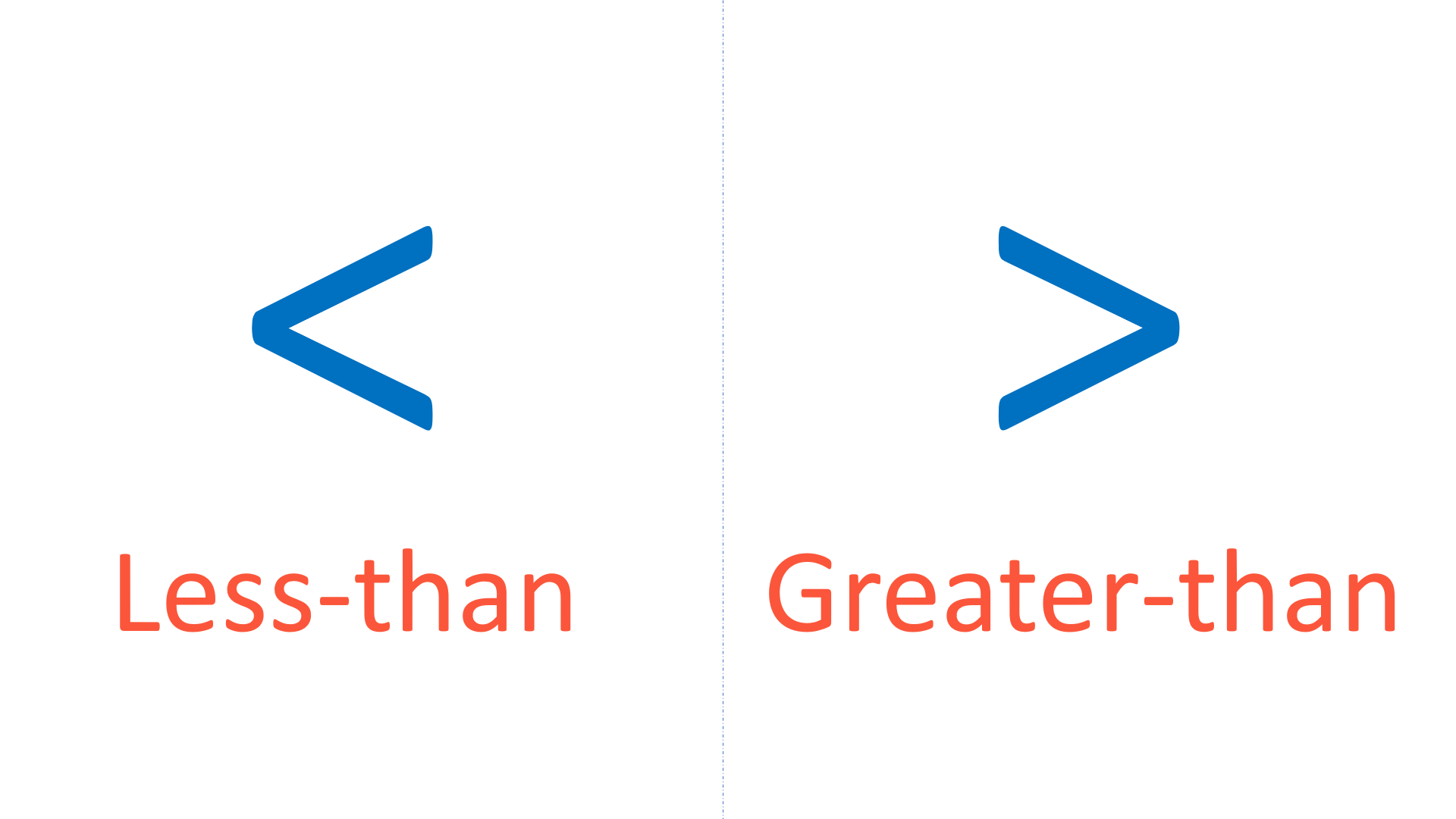Each sign is chosen in a number sentence so that the symbol points to the side that has the smallest value.

Each symbol then opens up to the side that has the greatest value.

We can use a number line to decide which side of a number sentence has the greatest value.

When teaching comparing number size, a number line is useful to help visualise the size of each value.

Here are the multiples of 10 from 0 to 100 shown on a number line.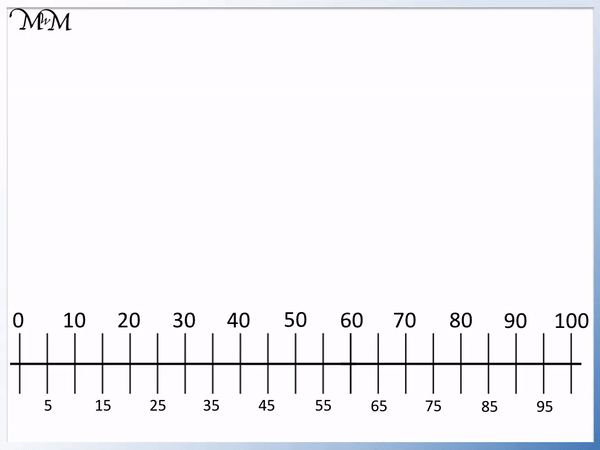When comparing number sentences at KS1 and KS2 (up to fourth grade), most children will be expected to use greater-than or less-than symbols for numbers up to 100.

In this example we have a missing symbol between 30 + 10 and 80.

We first evaluate the addition sum on the left of the missing symbol problem.

30 + 10 = 40.40 is less than 80 because it is further left on the number line.

We can use the less-than symbol ‘<' to write this mathematically.

We can think of the symbol like an arrow, pointing to the smaller value. We can also think of it as an open mouth opening up to the larger value.

We can write 40 < 80.

40 is the smaller number and so, the less-than symbol points at 40.

Because 40 < 80, we can also write 30 + 10 < 80.

Here is our next example of comparing number sentence values.

We have a missing symbol between 90 + 4 and 60.We first work out the value of 90 + 4.

90 + 4 = 94, which is to the right of 60 on the number line.

This means that 94 is greater than 60.

We use the greater-than symbol, ‘>’ to write this comparison mathematically.

60 is smaller than 94 and so, the arrow will point at 64. The ‘mouth’ will open up to the larger value of 94.

We can write 94 > 60 to say that 94 is greater-than 60.

Because 94 > 60, we can also write 90 + 4 > 60.

In this next example of comparing a subtraction sentence we have a missing symbol between 15 and 20 – 2.

We first evaluate the subtraction of 20 – 2.20 – 2 = 18, which is to the right of 15 on the number line.

18 is greater-than 15 and so the symbol opens to the 18 and points to the 15.

We can write 15 < 18 and so, 15 < 20 - 2.

In this next example we have a missing symbol between 12 + 14 and 26.

We first evaluate the addition of 12 + 14.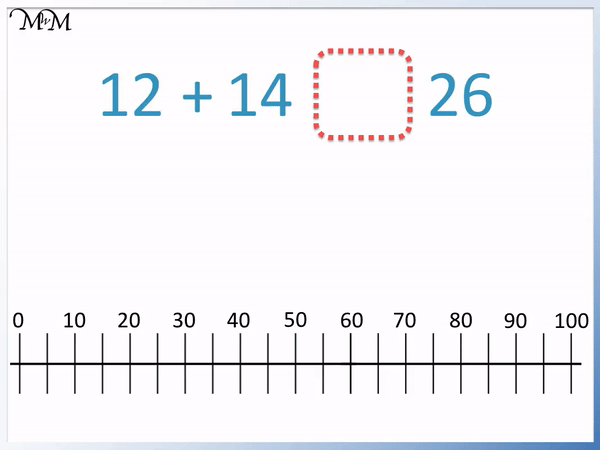12 + 14 = 26, which is the same as on the other side of the number sentence.

12 + 14 is the same value as 26 and so, we use an equals sign ‘=’ to show this.Now try our lesson on Rounding Off to the Nearest 10 on a Number Line where we learn how to round to the nearest 10.